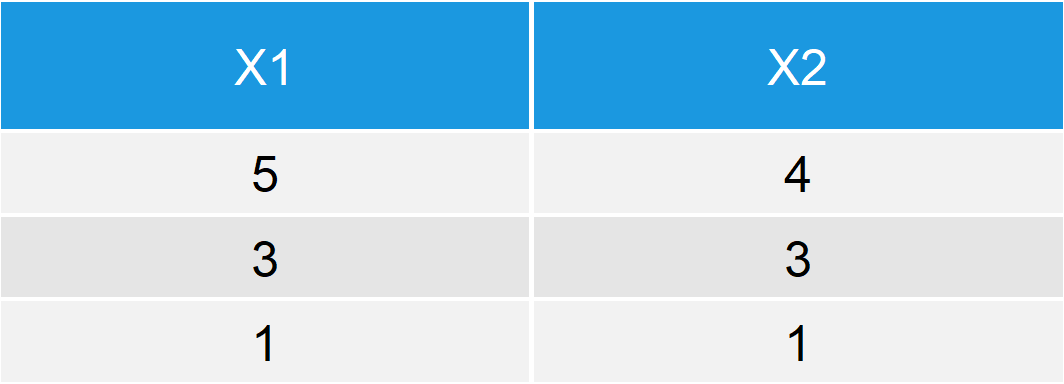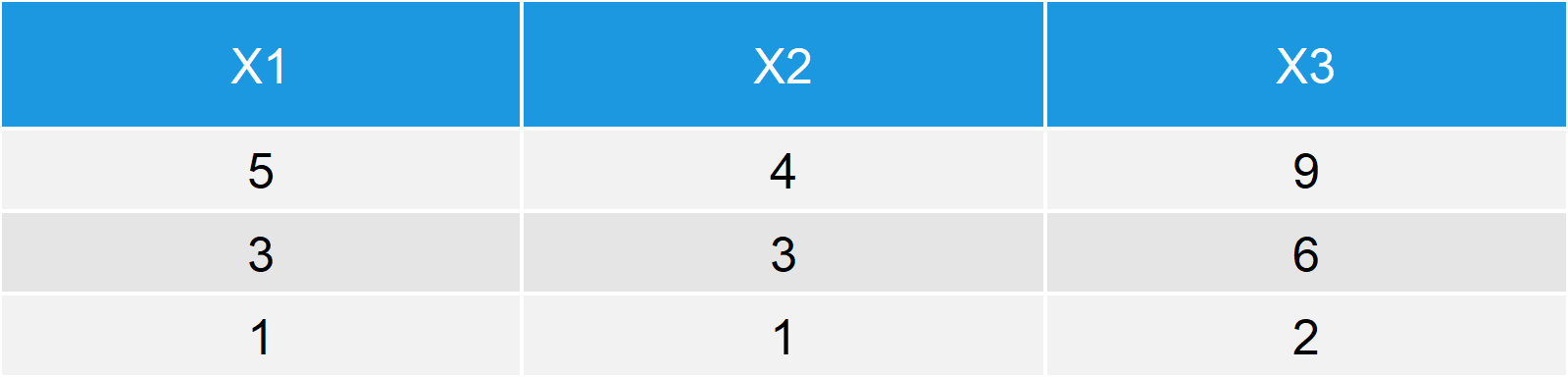# with & within Functions in R (2 Examples)

In this R programming tutorial I’ll show you how to use the with and within functions to handle data in R. Let’s first have a look at the basic R syntax and the definition of with() and within():

Basic R Syntax of with & within:

```with(data, x1 + x2)
within(data, x3 <- x1 + x2)```

Definition of with & within:

The with function evaluates an R expression in an environment constructed based on a data frame.

The within function evaluates an R expression in an environment constructed based on a data frame AND modifies the original data.

In the following article, I’ll explain based on two examples how to apply with and within in R. So let’s move on to the examples!

## Example 1: with Function in R

Before we can apply the with function, we need to create an example data frame in R:

```data <- data.frame(x1 = c(5, 3, 1),     # Create example data frame
x2 = c(4, 3, 1))
data                                    # Print data to RStudio console```Table 1: Example Data Frame in R.

Table 1 illustrates how our example data looks like. The data has two columns and three rows which contain numeric values.

Based on the with function, we can now evaluate expressions which take the variables of our data into account. For instance, we could compute the sum of our two variables:

```with(data, x1 + x2)                     # Apply with function
# 9 6 2```

Note: Since we used the with function, we didn’t have to call the data before using x1 and x2. This can make the handling of our data much easier, especially when many variables are involved in our expressions.

So what’s the difference of with and within? Let’s move on to the second example…

## Example 2: within Function in R

Based on the within function we can modify our data directly. Let’s assume we want to add a third column to our data, which contains the sum of the first and the second columns (i.e. x1 + x2). Then we can simply use the within function as follows:

`within(data, x3 <- x1 + x2)             # Apply within function`Table 2: Data After Applying within() Function in R.

As Table 2 shows: We just created a new data frame which contains our original data AND a new variable x3.

## Alternatives to with & within

R provides numerous functions for the handling of data. Similar functions to with and within are, e.g., attach & detach or transform.

If you want to learn more about data manipulation in general, I can also recommend the following video of the deltaDNA YouTube channel. Enjoy the video and let me know in the comments in case you have any questions or any feedback on the tutorial!

Please accept YouTube cookies to play this video. By accepting you will be accessing content from YouTube, a service provided by an external third party.If you accept this notice, your choice will be saved and the page will refresh.

Subscribe to the Statistics Globe Newsletter

•BrianOdhiambo
October 27, 2021 9:56 pm

Hi,which packagé do I need to load so as use thé with( ) fonction.

•October 28, 2021 7:18 am

Hey Brian,

You don’t have to install any packages, since the with() function is already provided by Base R.

Regards,
Joachim

••December 15, 2021 7:39 am